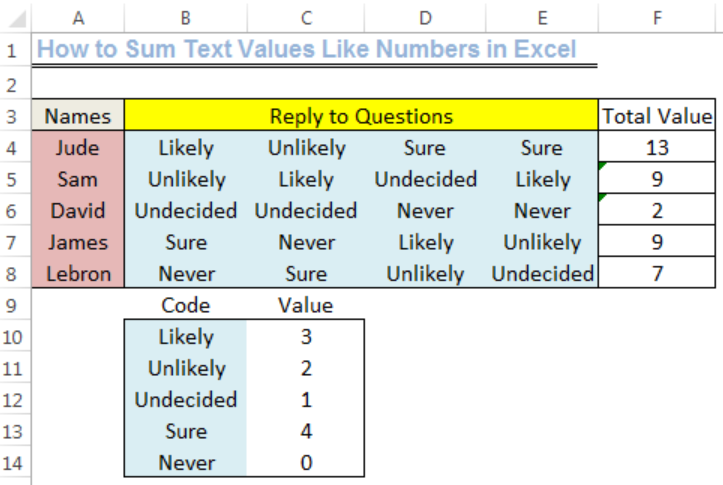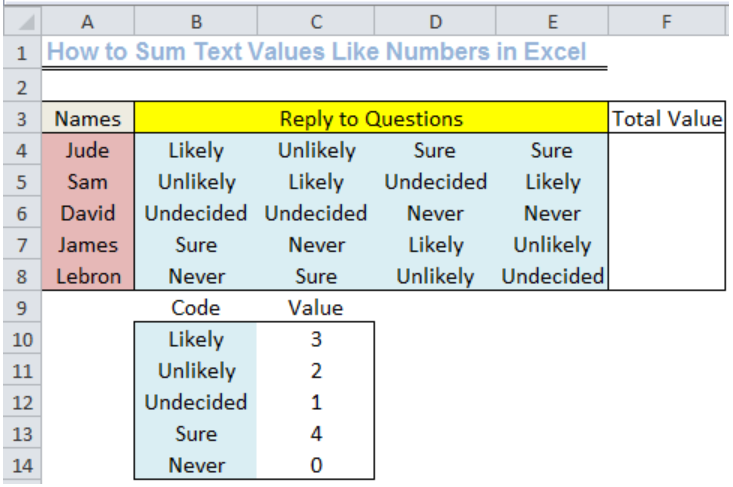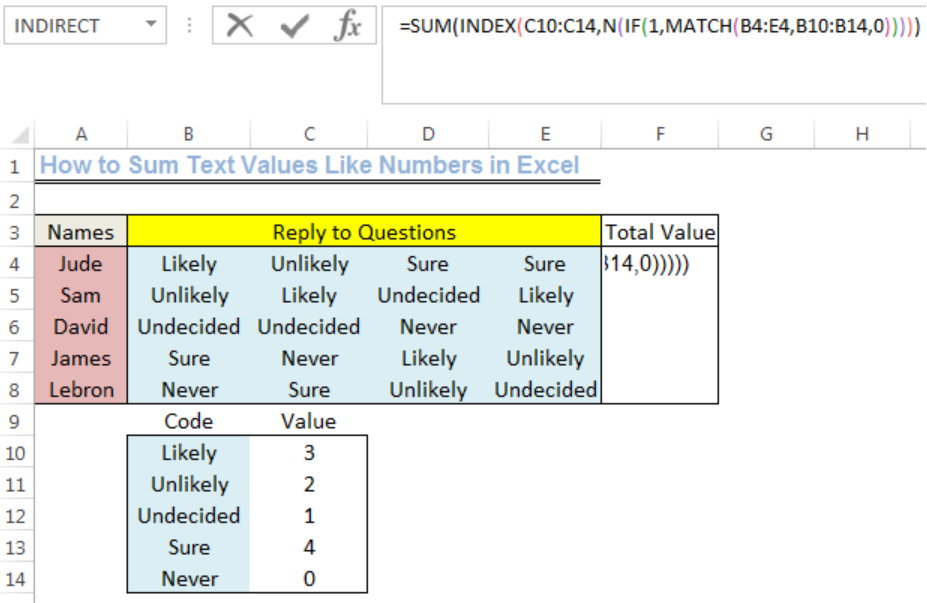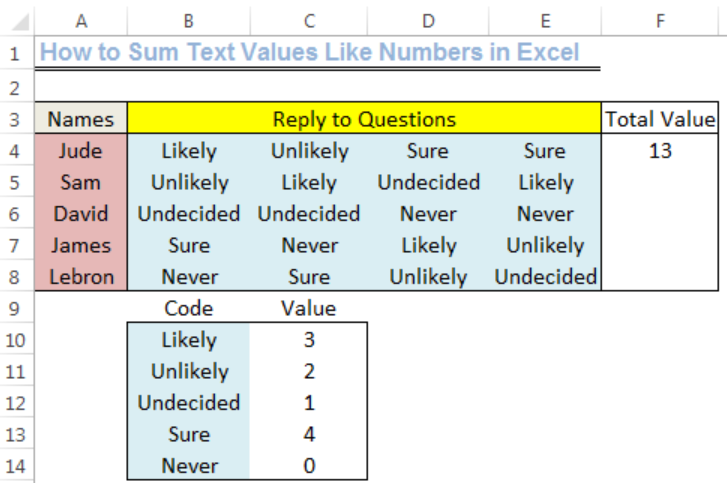Get instant live expert help with Excel or Google Sheets“My Excelchat expert helped me in less than 20 minutes, saving me what would have been 5 hours of work!”

#### Post your problem and you'll get expert help in seconds

Your message must be at least 40 characters
Our professional experts are available now. Your privacy is guaranteed.

# Learn How to Sum Text Values Like Numbers in Excel

We can sum text values as numbers after we have ASSIGNED NUMBERS to the Values. We can do this with the INDEX, MATCH, and SUM functions. This is useful for the evaluation of a questionnaire. The steps below will walk you through the procedure.Figure 1: How to Sum Text Values As Numbers

## Setting up the Data

• We will input the names of five students as shown in figure 2 into Column A from Cell A4 to Cell A8
• We will input their replies to the questions in Cell B4 to Cell E8 as shown in figure 2
• Cell F3 will be named as TOTAL VALUE
• We will assign numbers to each of the replies as shown in Column B from Cell B10 to Cell B14. The numbers are in Cell C10 to Cell C14Figure 2: Setting up the Data

## General Formula

`=SUM(INDEX(Value,N(IF(1,MATCH(Code,0)))))`

## Explanation

The functions that enable the translation of the ALPHABETS or TEXTS to NUMBERS are the INDEX and MATCH functions. The N and IF functions are required to ensure that we get results for the entire row. A shorter stream of functions will result in producing values for just a set of alphabets or text.

## Sum Text Values As Numbers

We will sum the reply of Jude by doing the following:

• We will click on Cell F4 and input the formula below:

`=SUM(INDEX(C10:C14,N(IF(1,MATCH(B4:E4,B10:B14,0)))))`Figure 3: Sum Text Values As Numbers

• We will press the following keys to get the result, CRTL+SHIFT+ENTERFigure 4: Sum Text Values As Numbers

## Instant Connection to an Expert through our Excelchat Service

Most of the time, the problem you will need to solve will be more complex than a simple application of a formula or function. If you want to save hours of research and frustration, try our live Excelchat service! Our Excel Experts are available 24/7 to answer any Excel question you may have. We guarantee a connection within 30 seconds and a customized solution within 20 minutes.

### Did this post not answer your question? Get a solution from connecting with the expert.Another blog reader asked this question today on Excelchat:
Related blogs## Subscribe to Excelchat.coAnother blog reader asked this question today on Excelchat: